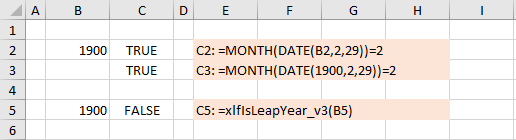# VBA is year a leap year

## 0. Quick guide - test for leap year

In this module:

1. Leap year function - from first principles
2. Leap year function - using VBA functions: DateSerial, Month, and Year

## 1. Leap year

A leap year is:

1. Any year that is exactly divisible by 4
1. except if exactly divisible by 100
1. but if exactly divisible by 400

The extra day is added to the month of February, with 29th February often described as a leap day. Exactly divisible means remainder 0 and can be determined by the VBA Mod operator.

The leap year calendar adjustment is needed because the earth rotates at 365.242375 times per year.

• Point 1 adds $+0.25$
• Then is corrected every 100 years in point 2 giving $-0.01$
• Finally point 3 adds 1 days every 400 year, equivalent to $+0.0025$.
• The result: $365 + 0.25 - 0.01 + 0.0025 = 365.2425$.

## 2. VBA code leap year identification

### 2.1 Using logic components

Code 1 and 2 are variations of the logic depicted in part 1 points 1 to 3. The VBA Mod operator divides two numbers and returns only the remainder.

Code 1: Function xlfIsLeapYear_v1 returns the Boolean value TRUE if Year is a leap year
Function xlfIsLeapYear_v1(Year As Integer) As Boolean
Dim bLY As Boolean
'  -- point 1 ---      --- point 2 ----     --- point 3 ----
If Year Mod 4 = 0 And (Year Mod 100 <> 0 Or Year Mod 400 = 0) Then bLY = True Else bLY = False
xlfIsLeapYear_v1 = bLY
End Function


Code 2 uses an If...Then construct to achieve the same outcome.

Code 2: Function xlfIsLeapYear_v2 returns the Boolean value TRUE if Year is a leap year.
Function xlfIsLeapYear_v11(Year As Integer) As Boolean
Dim Tmp As Boolean

If Year Mod 400 = 0 Then        ' point 3
Tmp = True
ElseIf Year Mod 100 = 0 Then    ' point 2
Tmp = False
ElseIf Year Mod 4 = 0 Then      ' point 1
Tmp = True
Else
Tmp = False
End If

xlfIsLeapYear_v2 = Tmp

End Function


VBA dates run from 1 January 100 to 31 December 9999 with corresponding serial date values -657434 to 2958465

Excel dates run from 1 January 1900 to 31 December 9999 with corresponding serial date values 1 to 2958465. Negative serial dates are not recognised in Excel. It is well known that the serial date sequence is incorrect because Excel includes 1900 as a leap. Thus 29th February 1900 with serial date value 60 is an error (inherited from Lotus 123). The VBA based functions in code 1, 2 and 3 each handle the 1900 date error correctly.

Table 1: A comparison of WS and VBA dates

Date Excel WS VBA
1 January 100 ######## -657434
30 December 1899 ######## 0, stored as 12:00:00 AM
31 December 1899 ######## 1
0 January 1900, WS date format applied to zero 0
1 January 1900 1 2
2 January 1900 2 3
28 February 1900 59 60
29 February 1900 (the 1900 leap year error) 60 61, stored as 1 March 1900
1 March 1900 61 61
31 December 2030 47848 47848

### 2.2 Using the VBA date functions

This is another variation uses VBA functions DateSerial, Month, and Year.

Code 3: Function xlfIsLeapYear_v3 returns the Boolean value TRUE if Year is a leap year
Function xlfIsLeapYear_v3(Year As Integer) As Boolean
xlfIsLeapYear_v3 = Month(DateSerial(Year, 2, 29)) = 2
End Function


Note: the WS version of code 3 would fail in the year 1900. See lines 2 and 3 of the worksheet in figure 1.Fig 1: Leap year WS formulas - with 1900 date error test data

WS formula line 3 =MONTH(DATE(1900,2,29))=2 returns TRUE

WS formula called from VBA Application.ExecuteExcel4Macro("MONTH(DATE(1900,2,29))=2") returns FALSE

• Development platform: Office 365 ProPlus Excel 64 bit.
• Published: 29th February 2016
• Revised: Saturday 27th of July 2019 - 12:50 AM, Pacific Time (PT)